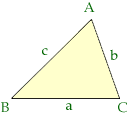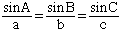Name: nikki Who is asking: Student Level: Secondary Question: Not sure how to figure this out - Find the measure, to the nearest degree, of each angle of a triangle with sides of the given lengths. 26, 35, 40 Hi Nikki, Two of the identities in trigonometry that are very useful and worth remembering are the Law of Sines and the Law of Cosines.In the diagram above a is the length of the side BC, b is the length of CA and c is the length of AB. Law of Sines: For any triangle ABCLaw of Cosines For any triangle ABC a2 = b2 + c2 - 2bc cos A In your problem you have the three side lengths and you are looking for the angles. In this case the Law of Cosines is appropriate since knowledge of a, b and c will allow you to solve for the angles. In particular if a = 26, b = 35 and c = 40 then 262 = 352 + 402 - 2(35)(40) cos A Solve for A. Cheers Harley Go to Math Central